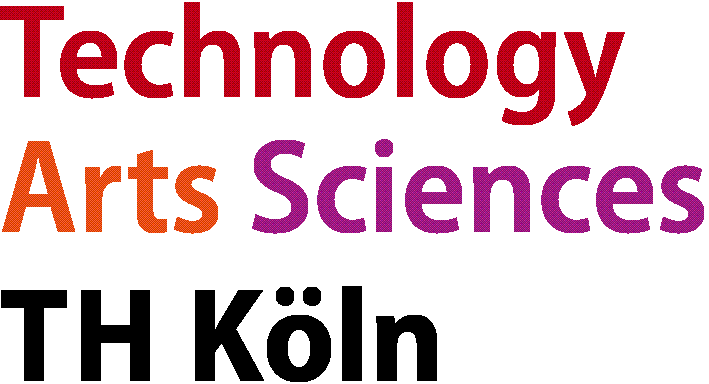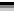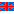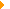Master of Eng. in Automation & ITDeutschEnglischAutomation & ITCourseModulesModelling and Simulation

# Modelling and Simulation of Technical Systems

Qualification aims

Students will comprehend methods and technologies of modelling and simulation of continuous and discrete event systems and develop their own small simulators. In particular, they will intensively work with "state of the art“ continuous and discrete event simulation software.

Students can

• model and simulate continuous systems
• model and simulate discrete event systems
• model data based systems (big data)
• model and simulate electrical energy systems

by

• understanding and applying the concepts of modelling of continuous systems, discrete event systems, data based systems, and electrical energy systems
• understanding the concepts of continuous and discrete event simulators
• applying modelling and simulation concepts to real-world technical problems
• designing simulation models of technical systems
• evaluating the quality of the simulation models
• performing simulation experiments
• using tools from statistical learning for modeling and understanding complex data sets
• applying standardized processes (e.g., the CRISP-DM process) to investigate complex data
• summarizing results in reports
• presenting results in oral presentations

to

• develop a deep understanding of the behavior of technical systems
• analyze and understand data from these systems
• be able to carry out design tasks such as system or plant design, controller design, etc. with the aid of simulation tools
• be qualified for a professional career as automation engineer

Courses

The module consists of 4 courses:

Modelling and Simulation of Continuous Systems

Tutor

Prof. Scheuring

Credit points

4 CP

Term

Spring

Contents

• Modelling of mechanical systems and process-engineering systems (thermodynamics, data on chemical media, valves, pumps, reactors, distillation columns, etc.)
• Design and organization of a simulator
• Sequential-modular simulation
• Dynamic simulation
• Introduction to UniSim
• Process industry applications

Modelling and Simulation of Discrete Event Systems

Tutor

Prof. Scheuring

Credit points

3 CP

Term

Spring

Contents

• Specifications of discrete event systems
• Compositional modelling of discrete event systems
• Object-oriented simulation of discrete event systems
• Introduction to Plant Simulation (formerly Simple ++, eM-Plant)
• Probability distribution
• Queuing theory
• Process and production industry application examples

Data-driven Modelling and Model Optimization

Tutor

Prof. Bartz-Beielstein + Prof. Konen

Credit points

5 CP

Term

Spring

Contents

• Data from real-world problems (industry, economy, science)
• Data preparation
• Linear regression, logistic regression
• Hypothesis testing
• Classification, linear discriminant analysis
• Tree-based methods
• Sequential parameter optimization (SPO)
• Model selection
• Treatment of missing values and huge data sets
• Data visualization
• Data mining, CRISP-DM Process
• Learning, especially advanced modelling techniques: Bootstrap, bagging, meta learner (e.g. random forests), empirical learning problems
• Evaluation of modelling results (e.g., error measures, overfitting, cross validation, precision and recall)

Modelling and Simulation of Electrical Energy Systems

Tutor

Prof. Freiburg

Credit points

2 CP

Term

Fall

Contents

• System requirements
• Electrical grids and grid components
• Grid operation
• Transmission line theory
• Stability aspects
• Network planning
• Network simulation
• Distributed energy resources
• Smart grids
• Power quality
• Asset management

Bibliography

• Stoer, J., et.al.: Introduction to numerical analysis. ISBN 0-387-95452-X
• Kincaid, D., et.al.: Numerical analysis. ISBN 0-534-38905-8
• Brenan, K., et.al.: Numerical solution of initial value problems in differential algebraic equations, 99 TLS 1067, ISBN 0-444-01511-6
• UniSim-Documentation, Honeywell 2006
• Kelton, W.D., Sadowski, R.P., Sadowski, D.A.: Simulation with Arena. McCraw-Hill 2002
• Banks, J.: Discrete-Event System Simulation, Prentice-Hall, 1996
• Liebl: Simulation. 2nd revision, Munich. Oldenbourg 1995
• Greasley A.: Simulation Modelling for Business. Ashgate Hants 2004.
• Feldmann K., Reinhardt G. (Hrsg.): Simulationsbasierte Planungssysteme für Organisation und Produktion. Springer Berlin 1999.
• Fishman G.S.: Discrete-Event Simulation. Springer Series in Operations Research. Springer 2001.
• Kelton, W.D., Sadowski, R.P., Sadowski, D.A.: Simulation with Arena. McGraw-Hill 2002
• Witten, I. H., Frank, E.: Data Mining, Hanser, 2nd ed., 2005.
• Hastie, T., Tibshirani, R., Friedeman, J.: The Elements of Statistical Learning. Springer, 2001.
• James, G., Witten, D., Hastie, T., and Tibshirani, R.: An Introduction to Statistical Learning with Applications in R. Springer, 4th edition, 2014.
• Law, A.M., Kelton, W.D.: Simulation Modeling and Analysis. McGraw-Hill, Boston. 2000.
• Bartz-Beielstein, T. et al.: Experimental Methods for the Analysis of Optimization Algorithms. Springer, 2010.
• Williams, G.: Data Mining with Rattle and R: The Art of Excavating Data for Knowledge Discovery. Springer, New York, 2011.
• Schwab, A. J.: Elektroenergiesysteme, Springer, 2006
• B. Buchholz, Z. Styczynski: Smart Grids, VDE Verlag, 2. Auflage 2018
• Oeding, D., Oswald, B. R.: Elektrische Kraftwerke und Netze, Springer Vieweg, 2016
• Quaschning, V.: Regenerative Energiesysteme, Hanser Verlag, 6. Auflage 2009
• Handschin, E.: Elektrische Energieübertragungssysteme, Hüthigverlag, 1992
• Heuck, K., Dettmann, K., Schulz, D.: Elektrische Energieversorgung, Springer, 2013
• Marenbach, R., et al.: Elektrische Energietechnik, Springer Vieweg, 2013
• Nelles, D.: Elektrische Energietechnik, Springer, 1998© 2021 TH Köln    Contact